www.hightopo.com

:: :: :: :: :: :::: ::194 隨筆 :: 1 文章 :: 412 評論 :: 0 引用

# 代碼實現（注：gif 的上傳大小有限，實際效果與還請參考 demo 鏈接）

gv.setMovableFunc(() => { return false }) // 禁止拖動
gv.getWireframe = (d) => { d.s('wf.visible', false) }  // 隱藏選中邊框
gv.setEye([583, -212, -789]) // 設置眼睛
gv.setCenter([-76, -654, -133]) // 設置中心點
gv.setFar(100000) // 設置遠端位置
gv.setNear(10) // 設置近端位置
gv.setInteractors([ new ht.graph3d.MapInteractor(gv) ]) // 設置交互限制
gv.setSkyBox(dm.getDataByTag('skyBox')) // 設置天空球
window.document.oncontextmenu = () => { return false } // 全局設置右鍵菜單禁用
gv.scene = { // 復制初始位置
eye: ht.Default.clone(gv.getEye()),
center: ht.Default.clone(gv.getCenter()),
far: ht.Default.clone(gv.getFar()),
near: ht.Default.clone(gv.getNear()),
}

gv.mi(e => {
let data = e.data
let kind = e.kind
if (kind === 'doubleClickBackground') { // 雙擊背景
gv.moveCamera(this.gv.scene.eye, this.gv.scene.center, {duration : 1000}) // 恢復視角
}
else if (kind === 'doubleClickData') { // 雙擊模型
gv.flyTo(data, {animation : {duration : 500}, distance : 800}) // 拉近視角
}
})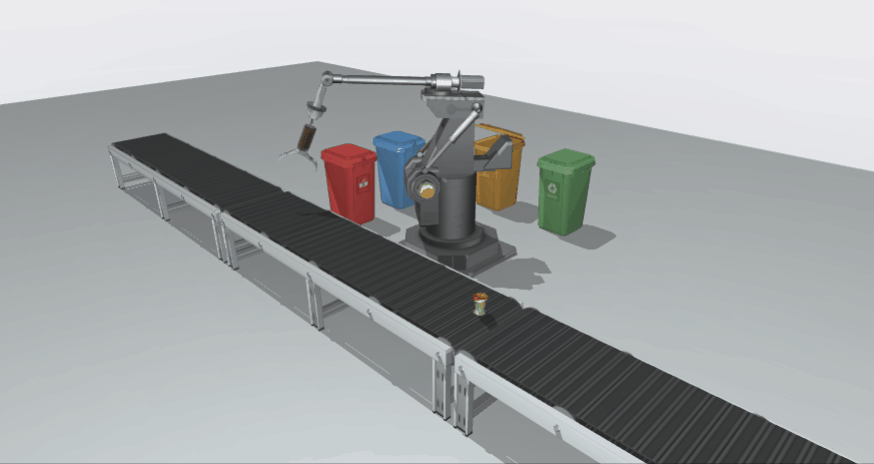function mechanicalArmAnim1() {
ht.Default.startAnim({
duration: 1000,
easing: (t) => { return t },
action: (v, t) => {
postbrachium.r3(degrees(0) + (degrees(20) - degrees(0)) * v, postbrachium.r3(), postbrachium.r3()) // 后臂向下移
},
finishFunc: () => {
setTimeout(() => {
mechanicalArmAnim2()
}, 300)
}
})
}
function mechanicalArmAnim2() {
ht.Default.startAnim({
duration: 1000,
easing: (t) => { return t },
action: (v, t) => {
postbrachium.p3(-208 + (-184 + 208) * v, postbrachium.p3(), postbrachium.p3()) // 后臂前伸
hydraulicRod1.r3(degrees(0) + (degrees(8) - degrees(0)) * v, hydraulicRod1.r3(), hydraulicRod1.r3()) // 液壓桿1傾斜
extensionRod1.r3(degrees(0) + (degrees(8) - degrees(0)) * v, extensionRod1.r3(), extensionRod1.r3()) // 伸長桿1傾斜
extensionRod1.p3(-169 + (-185 + 169) * v, -516 + (-511 + 516) * v, extensionRod1.p3()) // 伸長桿1伸長
hydraulicRod2.r3(degrees(0) + (degrees(-8) - degrees(0)) * v, hydraulicRod2.r3(), hydraulicRod2.r3()) // 液壓桿2傾斜
extensionRod2.r3(degrees(0) + (degrees(-8) - degrees(0)) * v, extensionRod2.r3(), extensionRod2.r3()) // 伸長桿2傾斜
extensionRod2.p3(-169 + (-185 + 169) * v, -516 + (-511 + 516) * v, extensionRod2.p3()) // 伸長桿2伸長
},
finishFunc: () => {
setTimeout(() => {
mechanicalArmAnim3()
}, 300)
}
})
}
function mechanicalArmAnim3() {
let oldValue = antebrachium.r3()
ht.Default.startAnim({
duration: 1000,
easing: (t) => { return t },
action: (v, t) => {
hydraulicRod1.r3(degrees(8) + (degrees(7) - degrees(8)) * v, hydraulicRod1.r3(), hydraulicRod1.r3()) // 液壓桿1傾斜
extensionRod1.r3(degrees(8) + (degrees(7) - degrees(8)) * v, extensionRod1.r3(), extensionRod1.r3()) // 伸長桿1傾斜
extensionRod1.p3(-185 + (-186 + 185) * v, -511 + (-507 + 511) * v, extensionRod1.p3()) // 伸長桿1伸長
hydraulicRod2.r3(degrees(-8) + (degrees(-7) - degrees(-8)) * v, hydraulicRod2.r3(), hydraulicRod2.r3()) // 液壓桿2傾斜
extensionRod2.r3(degrees(-8) + (degrees(-7) - degrees(-8)) * v, extensionRod2.r3(), extensionRod2.r3()) // 伸長桿2傾斜
extensionRod2.p3(-185 + (-186 + 185) * v, -511 + (-507 + 511) * v, extensionRod2.p3()) // 伸長桿2伸長
postbrachium.r3(degrees(20) + (degrees(25) - degrees(20)) * v, postbrachium.r3(), postbrachium.r3()) // 后臂向下移
antebrachium.r3(oldValue + (degrees(-40) - oldValue) * v, antebrachium.r3(), antebrachium.r3()) // 前臂向下移
claw1.r3(degrees(-20) + (degrees(-60) - degrees(-20)) * v, claw1.r3(), claw1.r3()) // 上爪抓取
claw2.r3(degrees(-60) + (degrees(-30) - degrees(-60)) * v, claw2.r3(), claw2.r3()) // 下爪抓取
},
finishFunc: () => {
mechanicalArmAnim4()
}
})
}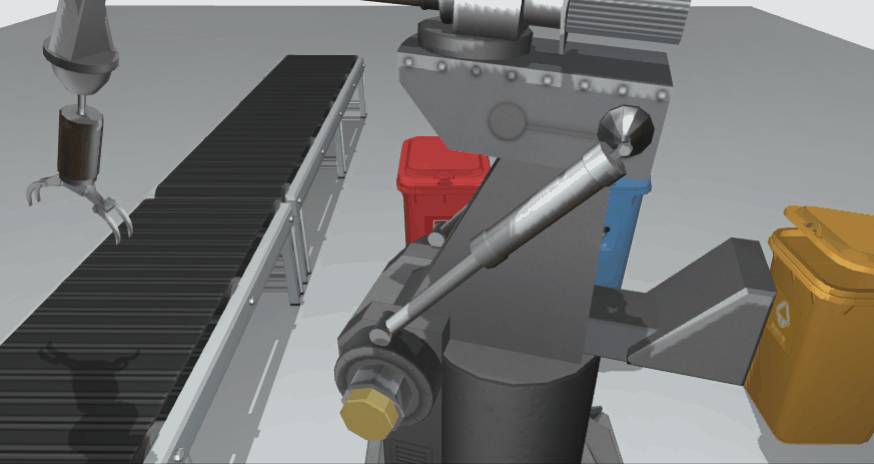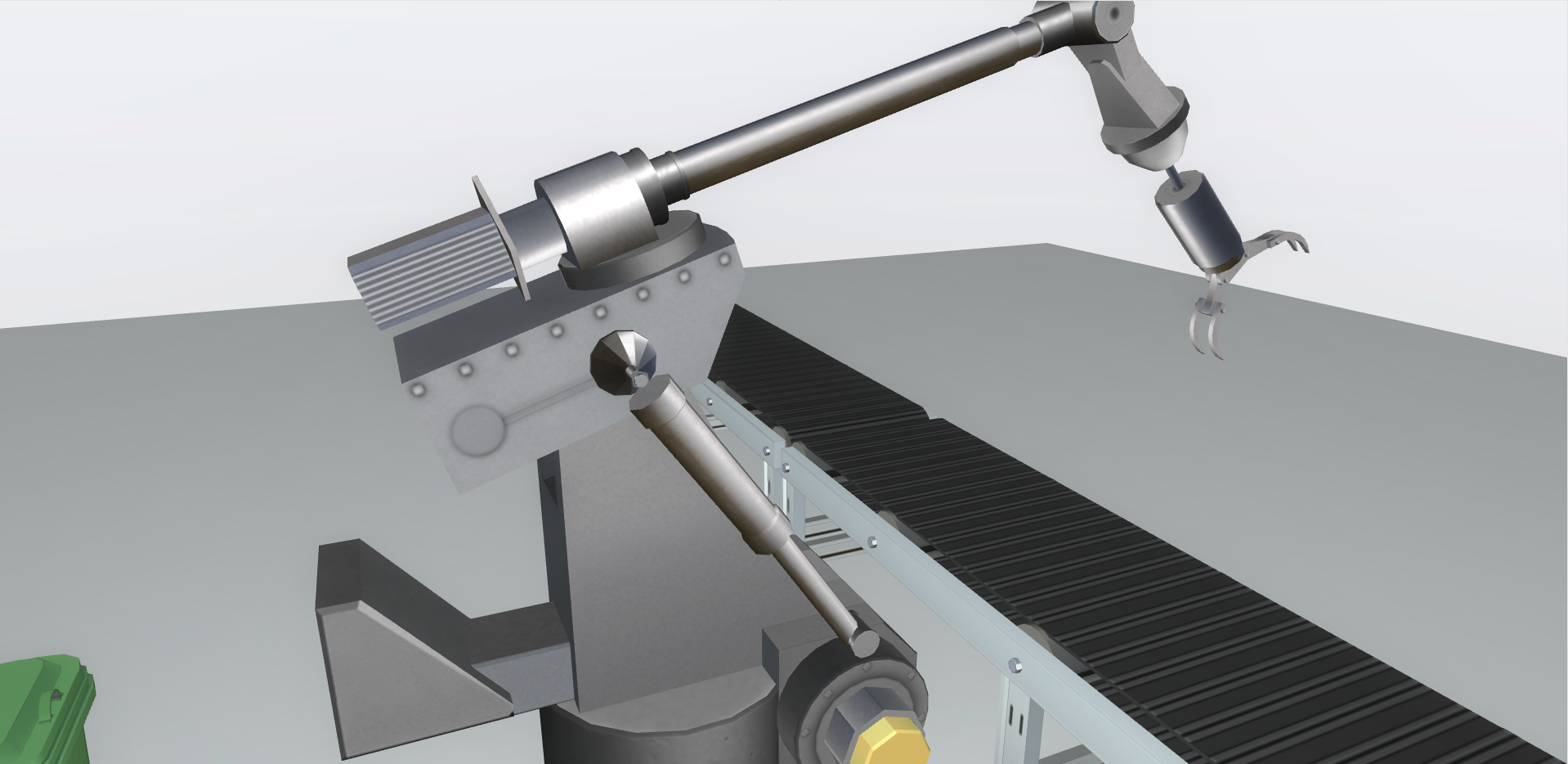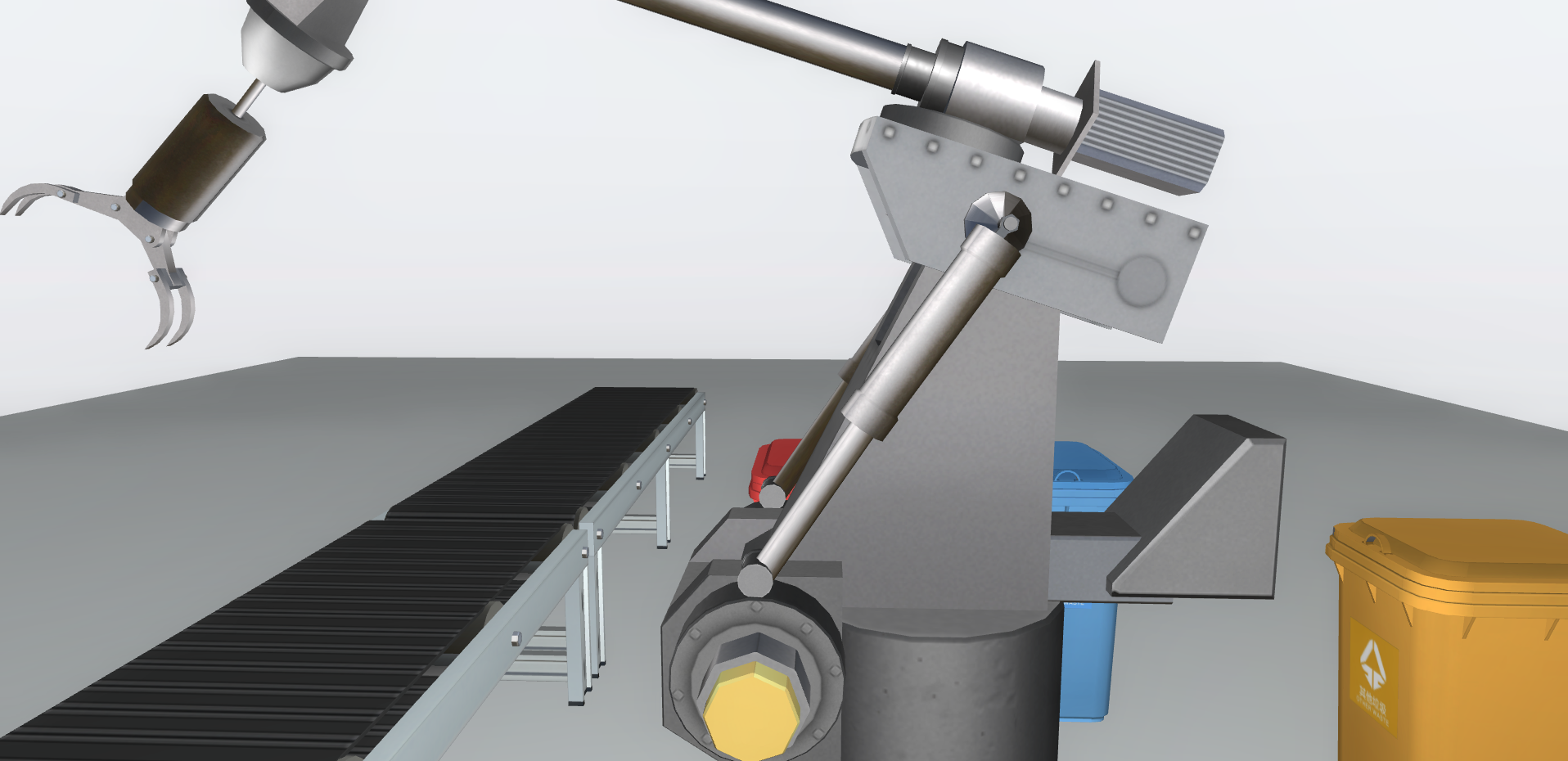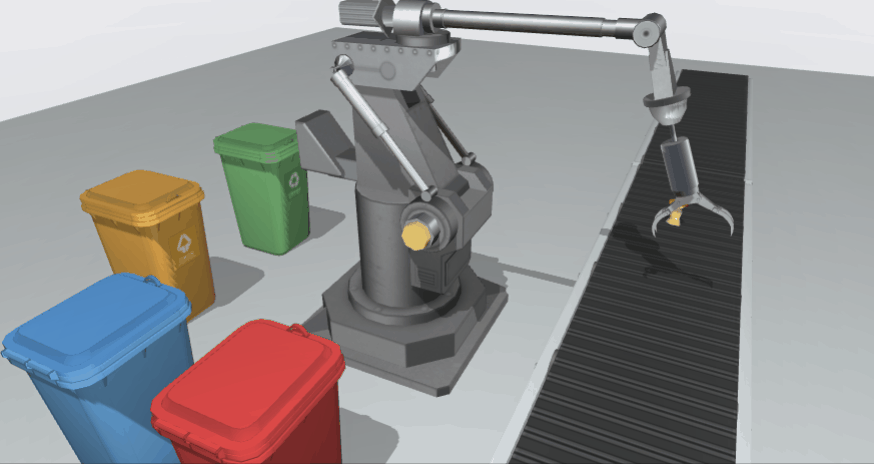gv.enableShadow(true, {
degreeX : 0,       // 投影 x 軸角度
degreeZ : -25,      // 投影 z 軸角度
intensity : 0.3,    // 陰影強度， 1 為黑色
quality : 'high',  // low / medium / high / ultra / 4096數值, 質量
type : 'soft',     // none / hard / soft
radius : 0.2,      // type 為 hard / soft 時，補充的邊緣厚度，用來提供更柔和的邊緣
bias : -0.003     // 深度浮點偏差補足
})

# 總結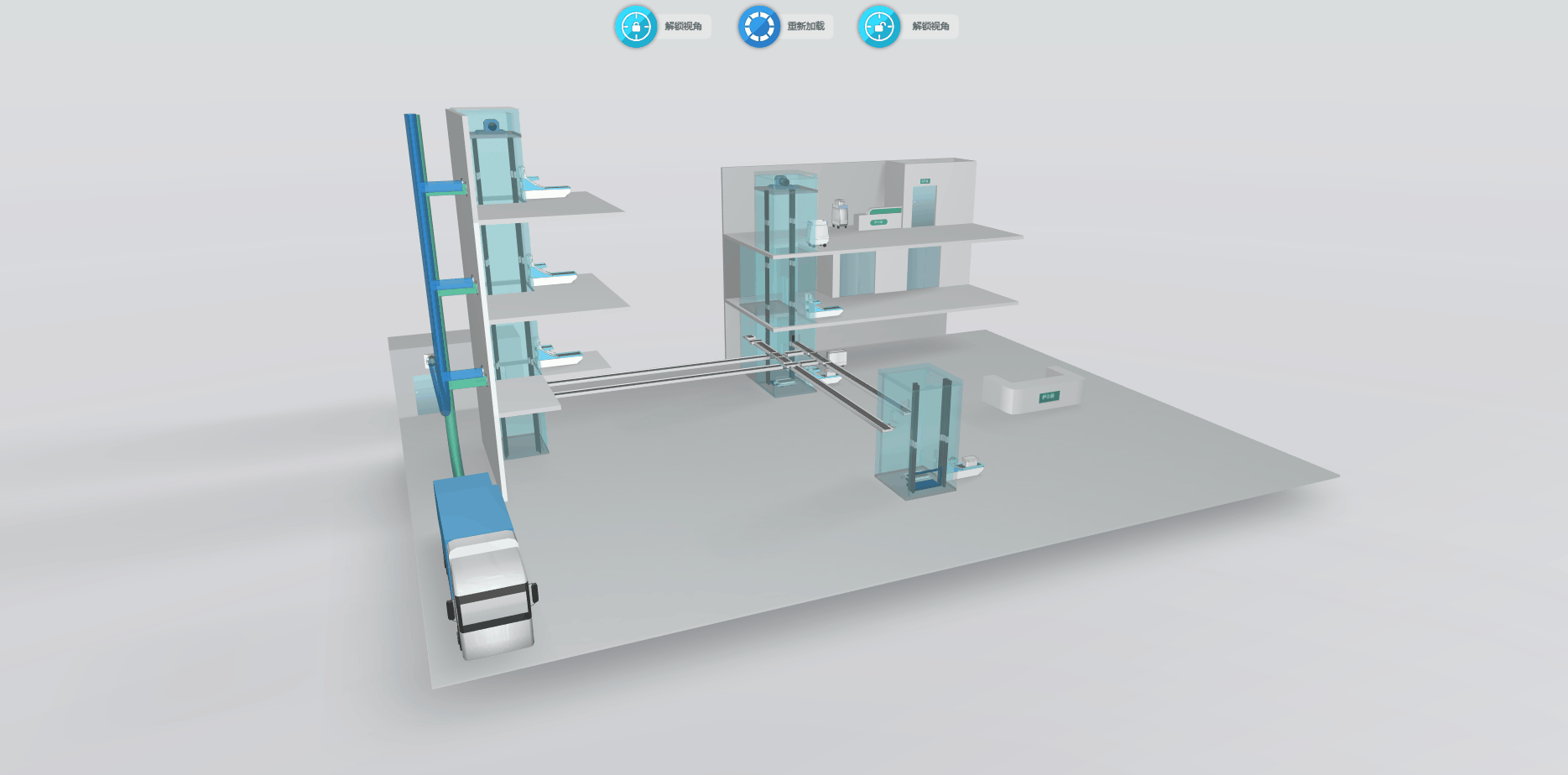posted on 2019-10-26 13:56  xhload3d  閱讀(...)  評論(... 編輯 收藏